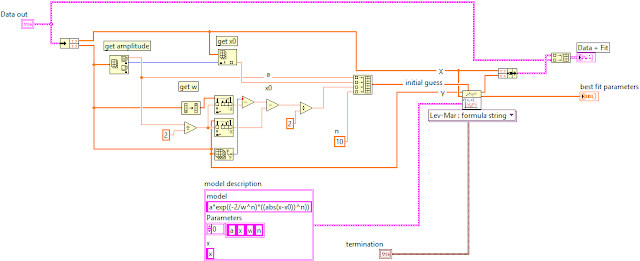## Sunday, January 17, 2016

### WE-FIT: A Tool to simplify Nonlinear data fitting

Fitting of data is a very common task when analyzing measured data. This involves finding a formula that best describes the relationship between one or more independent variables to a dependent variable. LabVIEW provides easy-to-use vis for a few commonly used formulae but often your data won’t be a good fit to any of these models. If that’s the case then more often than not you’ll want to use one of the Nonlinear Curve Fit VIs.

LabVIEW’s Nonlinear Curve Fit VIs include several fitting algorithms like the Levenberg-Marquardt algorithm (LM) and the trust-region dogleg algorithm (TRDL). The LM algorithm (which is a very popular and fast iterative fitting method) finds a local minimum, which means it does not always converge on an optimum (global) solution. The TRDL algorithm can be more robust in finding the global optimum solution, but is in general slower.

Whichever fitting algorithm is chosen, you’ll need to provide an initial estimation for each of the independent variables. Good initial estimates (often called guesses) are critical to achieving a good fit in reasonable time but this can be difficult to achieve, especially if the data is noisy. Figure 1 shows an example of a vi that fits to a Super Gaussian function. This implementation has some simple code to calculate the initial guesses but it is not very robust and will fail if the data is noisy (or for Super Gaussians with n<2). If the function becomes more complex the estimation of the initial guess might become more complex as well (e.g. the estimation of order of the Super Gaussian is such an example).Figure 1. A simple example of data fit to a Super Gaussian Function

The Wakefield Engineering fitting package for LabVIEW does the hard work of calculating the initial guesses for you (and choosing the best algorithm). The free version makes it very easy to fit to some of the most commonly used functions including Gaussian, Super Gaussian, Extreme, Boltzmann, Sine, Exponential Growth and Lorentzian. See Figure 2 for an example vi.Figure 2: Using the WE-FIT tool to fit data to a Sine Function

Not only does the Wakefield vi simplify fitting in LabVIEW, it also improves the robustness of fitting data that is “noisy”. In the package you will find examples, a handy template, and several useful analysis tools. Simply search for Wakefield in VI Package Manager to download. If you don’t see the function you need in the list contact us at ajg@wakefieldengineering.com for purchase of a pro version with additional functions.